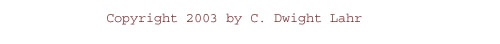Calculus on Demand at Dartmouth College## Lecture Web Pages

1. Modeling Discrete Data; Method of Least Squares
2. Functions and Their Graphs; New Functions from Old
3. Trigonometric Functions
4. Exponential and Logarithmic Functions
5. Case Study: Modeling the AIDS Data
6. Modeling Rates of Change
7. The Legacy of Galileo, Newton, and Leibniz; Limits of Functions; Limits at Infinity
8. Continuity; Tangent lines and Their Slopes
9. Tangent Lines and Their Slopes (contd.); The Derivative
10. Differentiation Rules
11. Derivatives of Trigonometric Functions
12. The Mean Value Theorem; Implicit Differentiation
13. Derivatives of Exponentials and Logs
14. Newton's Method; Linear Approximations
15. Antiderivatives and Initial Value Problems; Velocity and Acceleration
16. Case Study: Torricelli's Law
17. Modeling with Differential Equations; Separable Differential Equations: First Look
18. Exponential Growth and Decay; Separable Differential Equations
19. Slope Fields and Euler's Method; Case Study: Population Modeling
20. Issues in Curve Sketching
21. Modeling Accumulations
22. The Definite Integral; Properties of the Definite Integral
23. The Fundamental Theorem of Calculus; Techniques of Integration
24. Trapezoid rule; Areas Between Curves
25. Arc Length
26. Case Study: Flood Watch
27. Inverse Trigonometric Functions
28. Related Rates
29. Optimization
30. Volumes of Solids of Revolution

Calculus on Demand (COD) is an on-line version of a first calculus course. At Dartmouth, that course is called Math 3, Introduction to Calculus. COD contains lecture Web pages for the topics typically found in such a first course and in the College Board AP (AB) exam.

You can use COD to learn calculus for the first time, or to review material that you have already studied. COD is flexible in that you can begin with Lecture 1 and proceed in order through the lectures, or you can check your understanding of the things you think you know and concentrate on the topics where you need more work. Before you go any further, click on the Letter to Students, which explains how COD is organized and how to use it most effectively.

The lecture Web pages have been designed so that they can be used to study calculus with any textbook, although we specify a particular book so that we have something to refer to. If you already have a book, feel free to refer to it instead.

COD features the following on-line components, among others:

• Detailed examples with solutions.
• Video tutorials of problems being worked out step by step.
• Quizzes.
• Practice problems that tell you if you are right or wrong.
• Written solutions to the homework problems.
• Sample tests covering roughly the first third of the topics, the second third, and then comprehensive tests on the entire course.
• A bulletin board where you exchange information with other users of COD by posting comments or asking questions.

We hope you enjoy using COD to study calculus on-line.

Software requirements: For best results, use Mozilla Firefox. Click here to get Firefox and preferred software, all free.